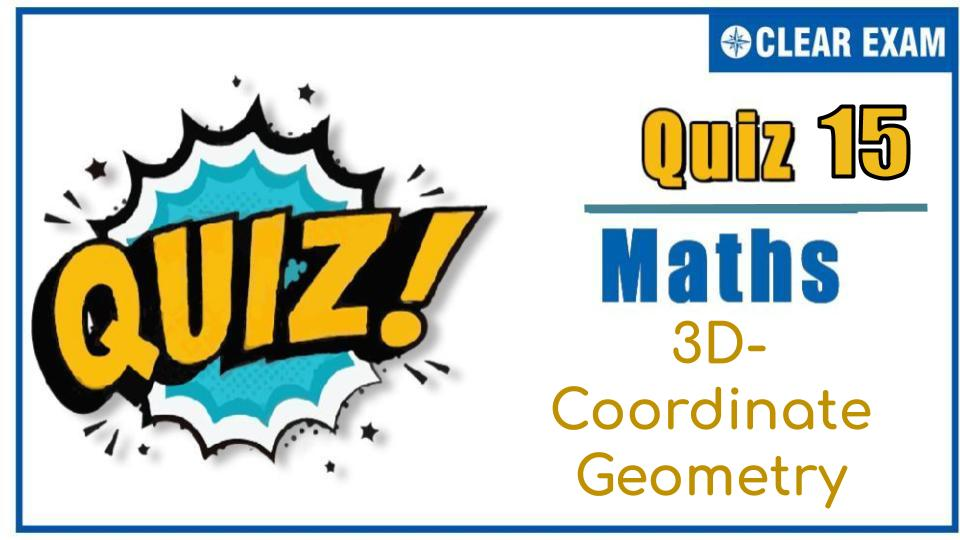## [LATEST]$type=sticky$show=home$rm=0$va=0$count=4$va=0

As per analysis for previous years, it has been observed that students preparing for JEE MAINS find Mathematics out of all the sections to be complex to handle and the majority of them are not able to comprehend the reason behind it. This problem arises especially because these aspirants appearing for the examination are more inclined to have a keen interest in Mathematics due to their ENGINEERING background. Furthermore, sections such as Mathematics are dominantly based on theories, laws, numerical in comparison to a section of Engineering which is more of fact-based, Physics, and includes substantial explanations. By using the table given below, you easily and directly access to the topics and respective links of MCQs. Moreover, to make learning smooth and efficient, all the questions come with their supportive solutions to make utilization of time even more productive. Students will be covered for all their studies as the topics are available from basics to even the most advanced..
3D Coordinate Geometry Quiz-15
Q1. Equation of a line passing through (-1,2,-3) and perpendicular to the plane 2x+3y+z+5=0 is:
•  $$\frac{(x-1)}{-1}=\frac{(y+2)}{1}=\frac{(z-3)}{-1}$$
•  $$\frac{(x+1)}{-1}=\frac{(y-2)}{1}=\frac{(z+3)}{1}$$
•  $$\frac{(x+1)}{2}=\frac{(y-2)}{3}=\frac{(z+3)}{1}$$
•  None of these
Solution
(c)
The required line passes through (-1,2,-3) and is perpendicular to the plane 2x+3y+z+5=0.
Therefore, it is parallel to the normal to the plane whose direction ratios are proportional to 2, 3, 1

Hence, direction ratios of the line are proportional to 2, 3, 1 and so its equation is
$$\frac{(x+1)}{2}=\frac{(y-2)}{3}=\frac{(z+3)}{1}$$

Q2.The equation of the plane passing through (1, 1, 1) and (1, -1,-1) and perpendicular to 2x-y+z+5=0 is:
•  2x+5y+z-8=0
•  x+y-z-1=0
•  2x+5y+z+4=0
•  x-y+z-1=0
Solution
(b) Any planes passing through (1,1,1) is
a(x-1)+b(y-1)+c(y-1)=0 …(i)
Since, it is passing through (1,-1,1), we get
a∙0+b(-2)+c(-2)=0
⇒0∙a-2b-2c=0
⇒0∙a+b+c=0 …(ii)
Eq. (i) is perpendicular to 2x-y+z+5=0 is
2a-b+c=0 …(iii)
From Eqs. (ii) and (iii), we get
a=b=1,c=-1

On substituting the value of a,b and c in Eq. (i), we get
x+y- z-1=0

Q3.  Ratio in which the xy-plane divides the join of (1, 2, 3) and (4, 2, 1) is:
•  3∶1 internally
•  3∶1 externally
•  1∶2 internally
•  2∶1 externally
Solution
(b)
Suppose xy-plane divides the join of (1, 2, 3) and (4, 2, 1) in the ratio λ∶1.
Then, the coordinates of the point of division are
$$\frac{(4λ+1)}{(λ+1)},\frac{(2 λ+2)}{(λ+1)},\frac{(λ+3)}{(λ+1)}$$
This point lies on xy-plane
∴z-coordinate =0⇒(λ+3)/(λ+1)=0⇒λ=-3
Hence, xy-plane divides the join of (1, 2, 3) and (4, 2, 1) externally in the ratio 3 : 1
ALTER We know that the XY-plane divides the segment joining P(x1,y1,z1) and Q(x2,y2,z2) in the ratio (-z1 ) ∶z2
∴XY-plane divides the join of (1, 2, 3) and (4, 2, 1) in the ratio -3∶1 i.e. 3∶1 externally

Q4. If the planes x+2y+kz=0 and 2x+y-2z=0, are at right angles, then the value of k is
•   2
•  -2
•  $$\frac{1}{2}$$
•  -$$\frac{1}{2}$$
Q5.The vector equation of plane passing through three non-collinear points having position vectors $\stackrel{\to }{a}$,$\stackrel{\to }{b}$,$\stackrel{\to }{c}$ is
•  $\stackrel{\to }{r}$∙($\stackrel{\to }{a}$×$\stackrel{\to }{b}$+$\stackrel{\to }{b}$×$\stackrel{\to }{c}$+$\stackrel{\to }{c}$×$\stackrel{\to }{a}$ )=0
•  $\stackrel{\to }{r}$×($\stackrel{\to }{a}$×$\stackrel{\to }{b}$+$\stackrel{\to }{b}$×$\stackrel{\to }{c}$ )=[$\stackrel{\to }{a}$ $\stackrel{\to }{b}$ $\stackrel{\to }{c}$]
•  $\stackrel{\to }{r}$∙($\stackrel{\to }{a}$×$\stackrel{\to }{b}$+$\stackrel{\to }{b}$×$\stackrel{\to }{c}$+$\stackrel{\to }{c}$×$\stackrel{\to }{a}$ )+[$\stackrel{\to }{a}$ $\stackrel{\to }{b}$ $\stackrel{\to }{c}$ ]=0
•  None of these
Solution
Not Avilable

Q6. The equation of the plane perpendicular to the line $$\frac{(x-1)}{1}=\frac{(y-2)}{-1}=\frac{(z+1)}{2}$$and passing through the point (2, 3, 1), is
•  $\stackrel{\to }{r}$∙($\stackrel{^}{i}$ +$\stackrel{^}{j}$ +2 $\stackrel{^}{k}$)=1
•  $\stackrel{\to }{r}$∙($\stackrel{^}{i}$-$\stackrel{^}{j}$+2 $\stackrel{^}{k}$)=1
• $\stackrel{\to }{r}$∙($\stackrel{^}{i}$-$\stackrel{^}{j}$+2 $\stackrel{^}{k}$)=7
•  None of these
Q7.The equation of the plane which bisects the line joining (2, 3, 4) and (6, 7, 8) is :
•  x-y-z-15=0
•  x-y+z-15=0
•  x+y+z-15=0
•  x+y+z+15=0
Q8. The angle between the lines $\stackrel{\to }{r}$=(4$\stackrel{^}{i}$-$\stackrel{^}{j}$)+s(2$\stackrel{^}{i}$+$\stackrel{^}{j}$-3$\stackrel{^}{k}$) and $\stackrel{\to }{r}$=($\stackrel{^}{i}$-$\stackrel{^}{j}$+2k ̂)+t($\stackrel{^}{i}$+3$\stackrel{^}{j}$+2k ̂) is:
•  3π/2
•  π/3
•  2π/3
•  π/6
Q9.The angle between the lines x=1,y=2 and y=-1,z=0 is:
•  30°
•  60°
•  90°
•
Q10. XOZ plane divides the join of (2, 3, 1) and (6, 7, 1) in the ratio :
•   3 : 7
•   2 : 7
•  -3∶7
•  -2:7## Want to know more

Please fill in the details below:

## Latest IITJEE Articles$type=three$c=3$author=hide$comment=hide$rm=hide$date=hide$snippet=hide ## Latest NEET Articles$type=three$c=3$author=hide$comment=hide$rm=hide$date=hide$snippet=hide

Name

ltr
item
BEST NEET COACHING CENTER | BEST IIT JEE COACHING INSTITUTE | BEST NEET & IIT JEE COACHING: 3D-Coordinate Geometry Quiz-15
3D-Coordinate Geometry Quiz-15
https://1.bp.blogspot.com/-FFUKuZp9h4E/X5G23RbgUGI/AAAAAAAAA4M/8cb3-AJalO44ktBn36iZHIvgzPuulo0ZgCLcBGAsYHQ/s0/Untitled%2Bpresentation%2B%252815%2529.jpg
https://1.bp.blogspot.com/-FFUKuZp9h4E/X5G23RbgUGI/AAAAAAAAA4M/8cb3-AJalO44ktBn36iZHIvgzPuulo0ZgCLcBGAsYHQ/s72-c/Untitled%2Bpresentation%2B%252815%2529.jpg
BEST NEET COACHING CENTER | BEST IIT JEE COACHING INSTITUTE | BEST NEET & IIT JEE COACHING
https://www.cleariitmedical.com/2020/10/3DCoordinateQuiz.html
https://www.cleariitmedical.com/
https://www.cleariitmedical.com/
https://www.cleariitmedical.com/2020/10/3DCoordinateQuiz.html
true
7783647550433378923
UTF-8

STAY CONNECTED